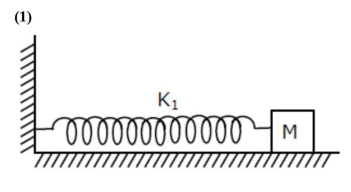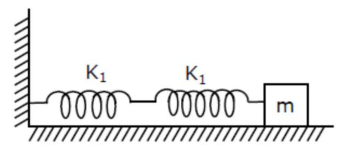# If two similar springs each of spring constantQuestion:

If two similar springs each of spring constant $\mathrm{K}_{1}$ are joined in series, the new spring constant and time period would be changed by a factor :

1. (1) $\frac{1}{2}, \sqrt{2}$

2. (2) $\frac{1}{4}, 2 \sqrt{2}$

3. (3) $\frac{1}{2}, 2 \sqrt{2}$

4. (4) $\frac{1}{4}, \sqrt{2}$

Correct Option: 1

Solution:$T=2 \pi \sqrt{\frac{m}{K_{1}}}$